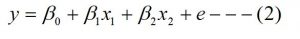# Multiple Regression Analysis

## Concept of Multiple Regression Analysis:

In simple regression analysis, the regression model is fit between one dependent and one independent variable to estimate the dependent variable or to measure the effectiveness of an independent variable on the dependent variable. When the regression model is fit between one dependent and more the one independent variable then this regression model is called the multiple regression model. In multiple regression analysis, the effect of the different independent variables on the dependent variable can be measured simultaneously.

Let ‘y’ be the dependent variable and x1, x2, x3 …………… xk be the ‘k’ independent variables. Then the multiple regression model is defined asWhere

y = dependent variable.
x1, x2, x3 …………… xk  are independent variables.
β0 = y-intercept.
β1 = Slope of y with variable x1 holding the remaining variables x2, x3 …,xk constant or Regression coefficient of y on x1 holding the remaining variables x2, x3 …………… xk constant.
β2 = Slope of y with variable x2 holding the remaining variables x1, x3 …,xk constant or Regression coefficient of y on x2 holding the remaining variables x1, x3 …………… xk constant.
β3 = Slope of y with variable x3 holding the remaining variables x1, x2, x4,  …,xk constant or Regression coefficient of y on x3 holding the remaining variables x1, x2, x4,  …,xk constant.
And so on. Similarly,
βk = Slope of y with variable xk holding the remaining variables  x1, x2, x3 …,xk-1 constant or Regression coefficient of y on xk holding the remaining variables x1, x2, x3 …,xk-1 constant.
e = error term or residual.

## Multiple Regression Model with Two Independent Variables:

Let ‘y’ be the dependent variable and x1 and x2 are two independent variables. The multiple regression model with two independent variables is defined as,Where

y = dependent variable.
x1and x2 are independent variables.
β0 = y-intercept.
β1 = Slope of y with variable x1 holding the remaining variable x2 constant or Regression coefficient of y on x1 holding the remaining variable x2 constant.
β2 = Slope of y with variable x2 holding the remaining variable x1 constant or Regression coefficient of y on x2 holding the remaining variable x1 constant.
e = error term or residual.

To fit the regression model (2), we have to estimate the value of β0, β1, and β2. To estimate the value of these parameters we use the principle of lest square. The following three normal equations of equation (2) can be obtained using the method of least square.

Now normal equations of equation (2) areOn solving these above three normal equations we can estimate the value of β0, β1, and β2. Hence the fitted multiple regression model isWhere,
The estimated value of the dependent variable for a given value of the independent variables.
β0 = y-intercept (or Estimated value of β0.)
b1 = Regression coefficients of y on x1 holding the effect of x2 constant (or Estimated
value of β1.)
b2 = Regression coefficients of y on x2 holding the effect of x1 constant (or Estimated
value of β2.)

## Interpreting the multiple regression coefficients:

Suppose we have the following multiple regression model1. The y-intercept β0 represents the average of the dependent variable when the value of independents variables are zero i.e. x1 = x2 =0. for example

Here β0 = 20, this means, the average value of dependent variable is 20 when x1 = x2 =0

2. The multiple regression coefficients b1 measure the average rate of increased or decreased in the value of a dependent variable (y) while         increasing the value of independent variable ‘x1’ by unit, by keeping the effect of another independent variable ‘x2’ constant. For example,

Here, β1 = 2.5, this means, the value of a dependent variable (y) is increased by 2.5 when the value of an independent variable (x1) is                 increased by 1, by keeping the effect of x2 constant.

3. The multiple regression coefficients β2 measure the average rate of increased or decreased in the value of a dependent variable (y)                     while increasing the value of independent variable ‘x2’ by unit, by keeping the effect of other independent variables ‘x1’ constant. For                 example,

Here, β2 = -3.8, this means, the value of the dependent variable (y) is decreased by 3.8 when the value of an independent variable (x2) is increased by 1, by keeping the effect of x1 constant.

## Error term or Residual:

The difference between the observed and estimated value of the dependent variable (y) is called error or residual and it is denoted by ‘e’

Wheree = Error term

y= Observed value of the dependent variable.= Estimated value of the dependent variable for a given value of a set of independent variables.

You may also like: Confidence Interval Estimate